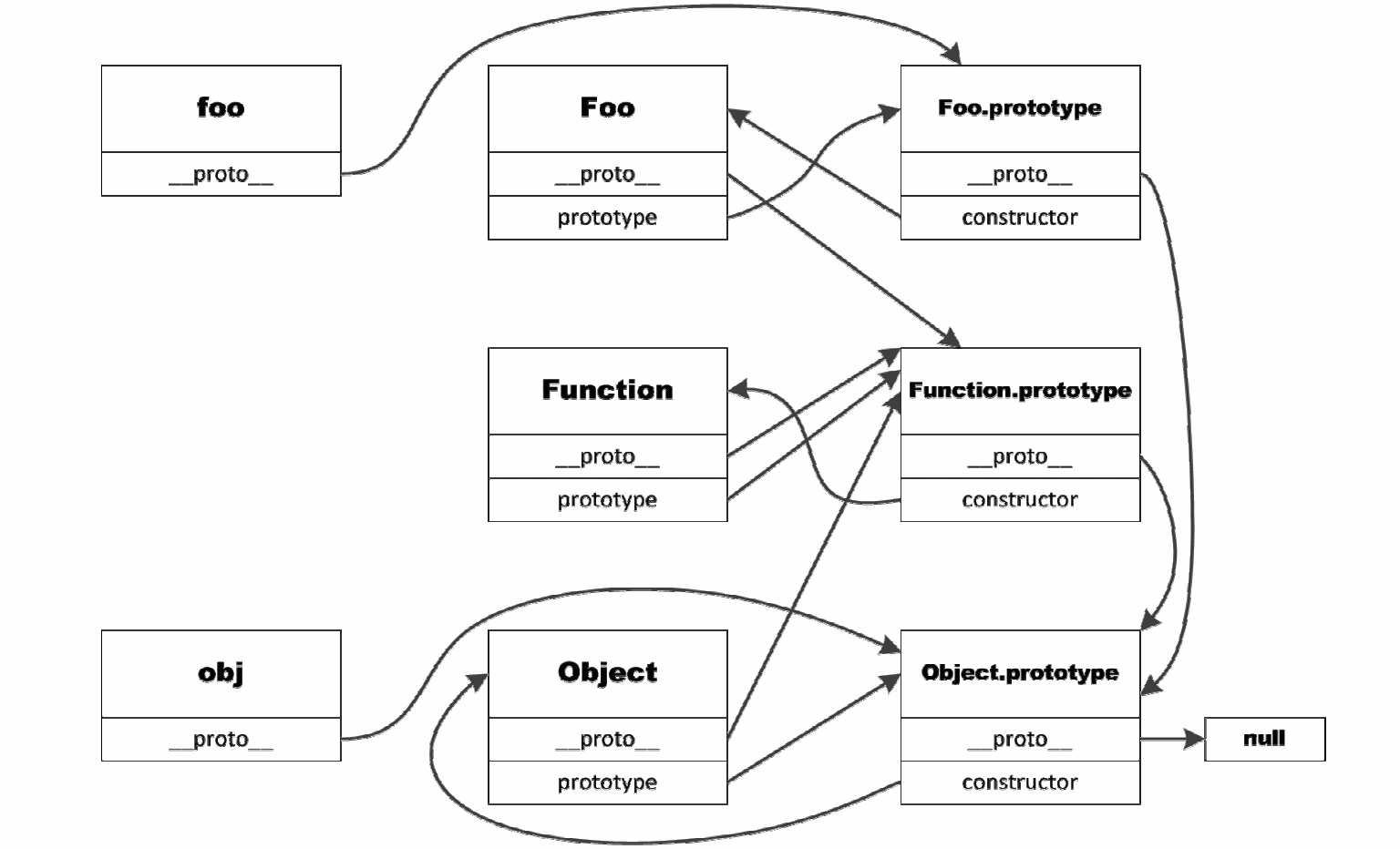# 理解JavaScript中的“面向对象”

## 二 “面向对象”的编程方式是如何演进而来的``````let radius = 2;
let pi = 3.14;
function area(){
}

console.log(area());
``````

``````let circle = {
pi:3.14,
s:function(){
}
}

console.log(circle.s());
``````

``````function Circle(radius) {
let circle = {};
circle.pi = 3.14;
circle.s = function() {
}
return circle;
}

var c1 = Circle(1);
var c2 = Circle(2);
console.log(c1.s());
console.log(c2.s());
``````

``````var prototype = {
s:function () {
}
}

let circle = {};
circle.pi = 3.14;
circle.s = prototype.s
return circle;
}

var c1 = Circle(1);
var c2 = Circle(2);
console.log(c1.s());
console.log(c2.s());
// true
console.log(c1.s === c2.s);
``````

``````var prototype = {
s:function () {
}
}

let circle = {};
Circle.pi = 3.14;
circle.s = prototype.s
return circle;
}
``````

``````function RedUnitCircle() {
let circle = {};
circle.parent = Circle(1);
circle.color = "red";
return circle;
}

var c3 = RedUnitCircle();
if (c3.s === undefined) {
console.log(c3.parent.s());
} else {
console.log(c3.s());
}
``````

## 三 this究竟指向哪里

1. 当函数被直接调用时，this指向golbal全局对象（node.js中），如果是在浏览器环境运行，则指向window对象。

``````function testFunc() {
console.log(this);
}
// global对象
testFunc();
``````

2. 当函数作为方法被调用时，this指向调用方法的对象。

``````var obj = {
name:"name",
log:function(){
console.log(this.name);
}
}
// name
obj.log();
``````

3. 当被作为构造函数被执行时，this指向新创建的对象。

``````function CreateObj() {
this.name = "name";
}
var obj = new CreateObj();
// name
console.log(obj.name);
``````

4. 使用bind，apply和call函数，能够显式的指定函数中的this指向。

``````var obj = {
name:"name"
}

function log(p) {
console.log(this.name, p);
}
function log2(p) {
console.log(this.name, p);
}
function log3(p) {
console.log(this.name, p);
}
// name Param
log.call(obj,'Param')
// name Param
log2.apply(obj,['Param']);
var newLog3 = log3.bind(obj);
// name Param
newLog3('Param');
``````

``````function create(){
return function() {
console.log(this);
}
}
function create2(){
return () => {
console.log(this);
}
}
var f = {name:"name", log:create(),log2:create2()};
//{name: 'name', log: ƒ, log2: ƒ}
f.log();
// global
f.log2();
``````

TypeScript或其他支持EX6的编译器也会将箭头函数编译成如下样式，非常清楚的描述了this在箭头函数中的指向：

``````function create2() {
var _this = this;
return function () {
console.log(_this);
};
}
``````

## 四 理解JavaScript中的面向对象

1. 创建一个空的对象，并让构造函数内的this指向此对象。

2. 将空对象的__proto__指向构造函数的prototype对象。

3. 执行构造函数，如果构造函数结果返回的是基本数据类型（包括无返回语句），则会被忽略，直接返回创建的对象。如果返回的是对象类型，则忽略之前创建的对象。

``````function People(name) {
this.name = name;
}

People.prototype.sayHi = function(){
console.log("Hello I am " + this.name);
}

var jaki = new People("Jaki");
// Hello I am Jaki
jaki.sayHi();
``````

``````function People(name) {
this.name = name;
}

People.prototype.sayHi = function(){
console.log("Hello I am " + this.name);
}

function Teacher(name, subject) {
People.call(this, name);
this.subject = subject;
}
// 修改子类原生指向
function TeacherPrototype(){
// 重写父类的方法
this.sayHi = function() {
// 调用父类方法
TeacherPrototype.prototype.sayHi.call(this);
// 子类自己的实现
console.log("教学："+this.subject);
}
}
// 子类原型设置
TeacherPrototype.prototype = People.prototype
Teacher.prototype = new TeacherPrototype()

var teacher = new Teacher("Jaki", "JavaScript");
//Hello I am Jaki
//教学：JavaScript
teacher.sayHi();

``````

## 五 再来深入理解下原型链

``````var a = {};
var b = new Object();
// {} {}
console.log(a, b);
// true
console.log(a.__proto__ === Object.prototype);
``````

``````var f = function(){
}
var f2 = new Function();
console.log(f, f2);
// true
console.log(f.__proto__ === Function.prototype);
``````

Object本身也是一个函数，因此Object的__proto__原型指向Function的prototype，同理，Function也是一种对象，因此Function的__proto__指向Object的prototype，在向上Object的prototype对象的__proto__就是null了，例如：

``````// true
console.log(Object.__proto__ === Function.prototype);
// true
console.log(Function.__proto__ === Function.prototype);
// true
console.log(Function.prototype.__proto__ === Object.prototype);
// null
console.log(Object.prototype.__proto__);
``````—— 珲少 QQ：316045346

{{o.name}}
{{m.name}}